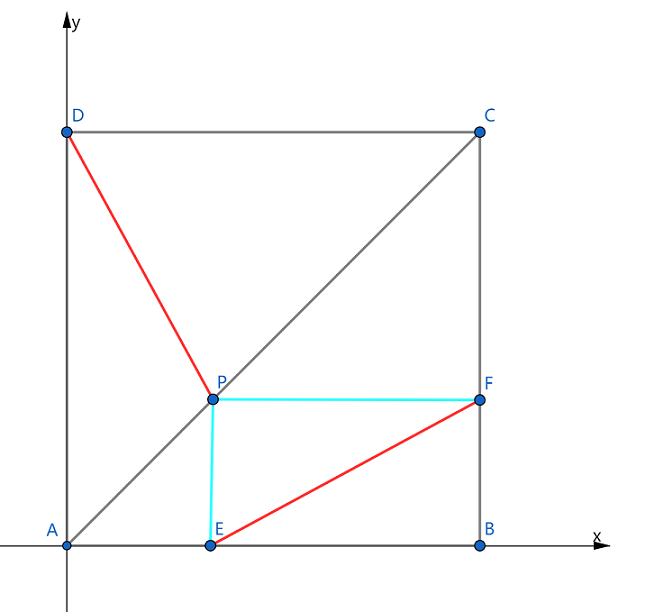安卓手机扫描二维码安装App﻿$A$﻿ 为原点，﻿$AB$﻿﻿$AD$﻿ 所在直线为﻿$x$﻿ 轴，﻿$y$﻿ 轴建立直角坐标系

﻿$\overrightarrow{DP} = (x,x-$﻿ ﻿$?1$﻿ ﻿$)$﻿

﻿$\overrightarrow{EF} =($﻿ ﻿$?2$﻿﻿$-x,x)$﻿

﻿$\overrightarrow{DP} \cdot \overrightarrow{EF} =0$﻿苹果手机扫描二维码安装App